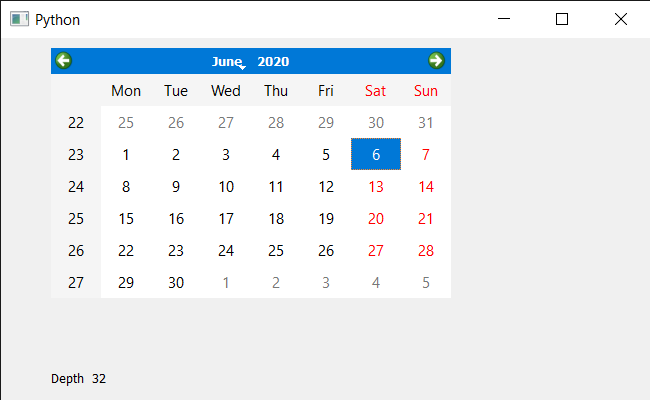Skip to content
Related Articles
PyQt5 QCalendarWidget – Bit Depth
• Last Updated : 09 Jun, 2020

In this article we will see how we can get depth of the QCalendarWidget. Color depth or color depth, also known as bit depth, is either the number of bits used to indicate the color of a single pixel, in this calendar, or the number of bits used for each color component of a single pixel.

In order to do this we will use `depth` method with the QCalendarWidget object.

Syntax : calendar.depth()

Argument : It takes no argument

Return : It return integer

Below is the implementation

 `# importing libraries``from` `PyQt5.QtWidgets ``import` `*` `from` `PyQt5 ``import` `QtCore, QtGui``from` `PyQt5.QtGui ``import` `*` `from` `PyQt5.QtCore ``import` `*` `import` `sys`` ` ` ` `class` `Window(QMainWindow):`` ` `    ``def` `__init__(``self``):``        ``super``().__init__()`` ` `        ``# setting title``        ``self``.setWindowTitle(``"Python "``)`` ` `        ``# setting geometry``        ``self``.setGeometry(``100``, ``100``, ``650``, ``400``)`` ` `        ``# calling method``        ``self``.UiComponents()`` ` `        ``# showing all the widgets``        ``self``.show()`` ` `    ``# method for components``    ``def` `UiComponents(``self``):`` ` `        ``# creating a QCalendarWidget object``        ``self``.calender ``=` `QCalendarWidget(``self``)`` ` `        ``# setting geometry to the calender``        ``self``.calender.setGeometry(``50``, ``10``, ``400``, ``250``)`` ` `        ``# setting cursor``        ``self``.calender.setCursor(Qt.PointingHandCursor)`` ` `        ``# creating a label``        ``label ``=` `QLabel(``self``)`` ` `        ``# setting geometry``        ``label.setGeometry(``50``, ``280``, ``420``, ``120``)`` ` `        ``# making it multi line``        ``label.setWordWrap(``True``)`` ` `        ``# getting depth``        ``value ``=` `self``.calender.depth()`` ` `        ``# setting text to the label``        ``label.setText(``"Depth  "` `+` `str``(value))`` ` ` ` `# create pyqt5 app``App ``=` `QApplication(sys.argv)`` ` `# create the instance of our Window``window ``=` `Window()`` ` ` ` `# start the app``sys.exit(App.``exec``())`

Output :Attention geek! Strengthen your foundations with the Python Programming Foundation Course and learn the basics.

To begin with, your interview preparations Enhance your Data Structures concepts with the Python DS Course. And to begin with your Machine Learning Journey, join the Machine Learning – Basic Level Course

My Personal Notes arrow_drop_up